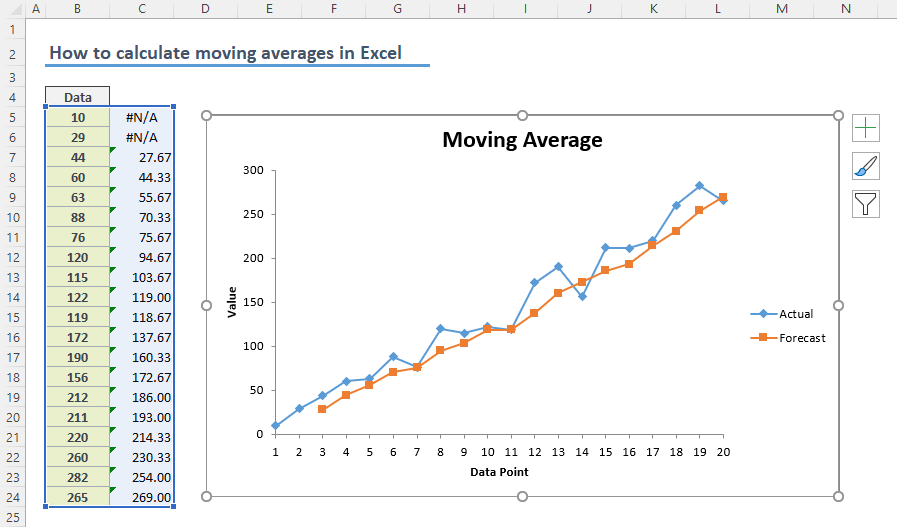## How to calculate simple moving average in Excel

Moving average calculations is a common way of smoothing data to reduce the effects of random, short-term fluctuations. In this guide, we’re going to show you how to calculate simple moving averages in Excel using three methods. Download Workbook What is Simple Moving...## How to calculate average by week number

Calculating averages by week number is a bit different than doing this by month. Instead of finding start and end dates of date range, we focus on the exact week number using the WEEKNUM function. Syntax =AVERAGEIFS( range of values to calculate average, range of week...## How to calculate average of values with OR operator

The AVERAGEIFS function can calculate the average of values with multiple conditions. However, these conditions are linked with AND operator. But if you want to handle AVERAGE OR combination, AVERAGEIFS function will not work. This article shows how to calculate...## How to calculate AVERAGE with intersecting tables

This article shows how to calculate average with intersecting tables using AVERAGE function and intersect operator, the space character (” “). Syntax =AVERAGE(first range second range) Steps Type =AVERAGE( function Select or type the first range reference...## How to calculate monthly averages

This article shows you how to calculate monthly averages from a data table containing dates and values using AVERAGEIFS and EOMONTH functions. If you only want to calculate the number of days in a month, please refer to the article: How to find number of days in month...